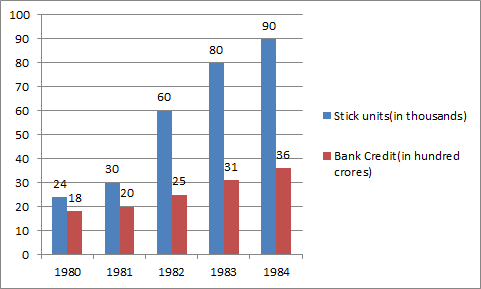# Data Interpretation - Bar Graph

>>>>>>>>Bar Graph

Instructions:

Study the graph carefully and answer the questions 51-55 given below• A

50• B

150• C

25• D

None of these• Option : D
• Explanation : Number of increase in 1981 to 1983 = 80-30 = 50

% = 50/30 * 100

= 166.66%

Instructions:

Study the graph carefully and answer the questions 51-55 given below

• A

Rs. 5 crore• B

Rs. 50 crore• C

Rs. 500 crore• D

Rs. 50 lac• Option : C
• Explanation : Required difference = (25-20) hundred crore

= 5 * 100 crore = 500 crore

Instructions:

Study the graph carefully and answer the questions 51-55 given below

• A

Rs. 3 crore• B

Rs. 3 lac• C

Rs. 30 lac• D

Rs. 4 lac• Option : B
• Explanation : Required bank credit per sick unit

= Rs. 10 * 100 crore/ 30 * 1000

=Rs. 10 * 1000000000/ 30 * 1000

=Rs. 3.33 Lakhs

=Rs. 3 lakhs

Instructions:

Study the graph carefully and answer the questions 51-55 given below

• A

1980• B

1981• C

1982• D

1983• Option : A
• Explanation : 1980 = 18 * 100 Crore / 24 * 1000 = Rs. 7.5 lakhs

1981 = 20 * 100 Crore / 30 * 1000 = Rs. 6.6 lakhs

1982 = 25 * 100 Crore / 60 * 1000 = Rs. 4.16 lakhs

1983 = 31 * 100 Crore / 80 * 1000 = Rs. 3.87 lakhs

1984 = 36 * 100 Crore / 90 * 1000 = Rs. 4 lakhs

Instructions:

Study the graph carefully and answer the questions 51-55 given below

• A

Rs. 32 crore• B

Rs. 71 crore• C

Rs. 71 lac• D

Rs. 4 lacRelated Quiz.
Bar Graph